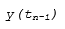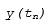## 比较求解器

### 定步长和变步长求解器

`ex_multirate` 示例模型显示了变步长求解器如何缩短多速率离散模型的仿真时间。```[0.0 0.25 0.5 0.75 1.0 1.25 1.5 ...] ```

```[0.0 0.5 0.75 1.0 1.5 ...] ```

### 显式与隐式连续求解器

`$\stackrel{˙}{x}=f\left(x\right)$`

`$F\left(\stackrel{˙}{x},x\right)=0$`

`$M\left(x\right).\stackrel{˙}{x}=f\left(x\right)$`

M(x) 称为质量矩阵，$f\left(x\right)$ 是外力函数。当您在模型中使用物理建模模块时，系统将变成线性隐式系统。

### 单步与多步连续求解器

Simulink 求解器库中提供了单步和多步求解器。单步求解器使用上一个时间点的解以及 tn 与 tn-1 之间的多个点的导数值来估算。这些点是子时间步。